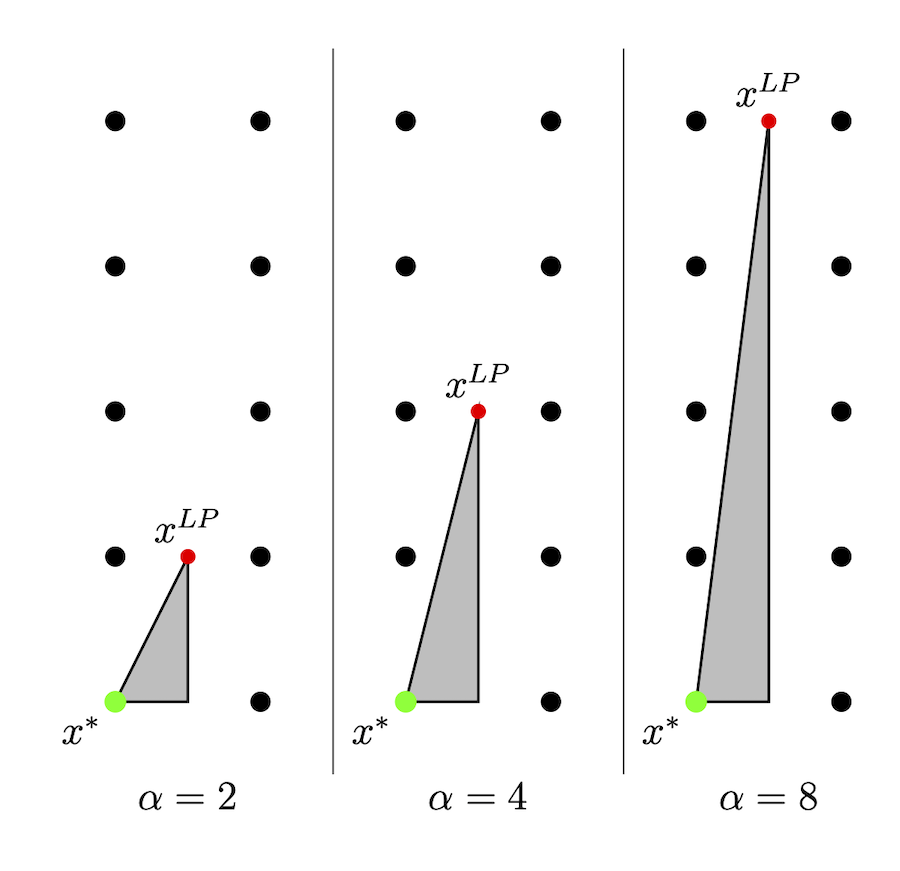•Gurobi Staff

Hi Ouafa,

The value of the root relaxation is completely dependent on the formulation given to the solver. It's nice to have a root relaxation value that is as close as possible to the true optimal objective value, as the root relaxation provides the initial bound on the optimal solution. The root relaxation value can be improved by "tightening" your formulation, i.e., reformulating the problem so the linear constraints form a smaller feasible region. How to do this depends on the model you're trying to solve. It could be as simple as using a tighter/smaller value of $$M$$ in big-$$M$$ constraints.

To try to improve the bound faster, you could try a more aggressive Cuts parameter or MIPFocus=3.

Also, note that the relaxation solution can be arbitrarily "bad" relative to the optimal solution. For example, consider the problem below for a parameter $$\alpha \geq 0$$:

\begin{alignat*}{2} \max && x_2 \qquad\quad & \\ \textrm{s.t. } && -\alpha x_1 + x_2 &\leq 0 \\ && x_1 \phantom{ + x_2}\ \,&\leq 0.5 \\ && x &\in \mathbb{Z}_+^2. \end{alignat*}

For all $$\alpha \geq 0$$, the feasible region of the above problem is comprised of the single point $$(0, 0)$$, and the optimal solution is $$z^* = 0$$. However, we can get very different relaxation solutions and optimal objective values ( $$x^{LP}$$ and $$z^{LP}$$, respectively) depending on the value of $$\alpha$$. Consider the feasible regions below for $$\alpha \in \{2, 4, 8\}$$:• For $$\alpha = 2$$, we have $$x^{LP}=(0.5,1)$$ and $$z^{LP}=1$$
• For $$\alpha = 4$$, we have $$x^{LP}=(0.5,2)$$ and $$z^{LP}=2$$
• For $$\alpha = 8$$, we have $$x^{LP}=(0.5,4)$$ and $$z^{LP}=4$$

More generally, the relaxation solution is $$x^{LP}=(0.5,\alpha/2)$$, with $$z^{LP}=\alpha/2$$. So, we can make the relaxation worse and worse by increasing $$\alpha$$.

Eli

•Hello Eli,

I guess you are right. Thanks for the prompt reply!

Ouafa